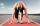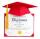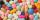# Masons

1 mason casts 30.8 meters square in 8 hours. How long casts 4 masons 178 meters square?

Result

x =  11.6 h

#### Solution:Leave us a comment of example and its solution (i.e. if it is still somewhat unclear...):

Showing 0 comments:Be the first to comment!## Next similar examples:

1. Runner 2Marian run 12 meters in 8 seconds. How far would Marian run for 70 seconds, if still run at the same pace?
2. DiplomasSuzanne helped write out diplomas. Issuance its first took 3 minutes, each next 2.3 minutes. How much time it will take to write out n=16 diplomas (including the first), write out this pace?
3. Greg and BillGreg is 18 years old. He is 6 less than 4 times Bill's age. How old is Bill?
4. Grandfather and grandmotherThe old mother is 5 years younger than the old father. Together they are 153 years old. How many years has each of them?
5. PillsIf it takes 20 minutes to run a batch of 100 pills how many minutes would it take to run a batch of 50 pills
6. Scale of the mapDetermine the scale of the map if the actual distance between A and B is 720 km and distance on the map is 20 cm.
7. TVsProduction of television sets increased from 3,500 units to 4,200 units. Calculate the percentage of production increase.
8. DonutsFind how many donuts each student will receive if you share 126 donuts in a ratio of 1:5:8
9. New refrigeratorNew refrigerator sells for 1024 USD, Monday will be 25% discount. How much USD will save, and what will be the price?
10. Percents - easyHow many percent is 432 out of 434?
11. The percentages in practiceIf every tenth apple on the tree is rotten it can be expressed by percentages: 10% of the apples on the tree is rotten. Tell percent using the following information: a. in June rained 6 days b, increase worker pay 500 euros to 50 euros c, grabbed 21 fro
12. Conference148 is the total number of employees. The conference was attended by 22 employees. How much is it in percent?
13. Down syndromeDown syndrome is one of the serious diseases caused by a gene mutation. Down syndrome occurs in approximately every 550-born child. Express the incidence of Down's syndrome in newborns at per mille.
14. Base, percents, valueBase is 344084 which is 100 %. How many percent is 384177?
15. Apples 2James has 13 apples. He has 30 percent more apples than Sam. How many apples has Sam?
16. Unknown numberIdentify unknown number which 1/5 is 40 greater than one tenth of that number.
17. EquationSolve the equation: 1/2-2/8 = 1/10; Write the result as a decimal number.